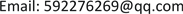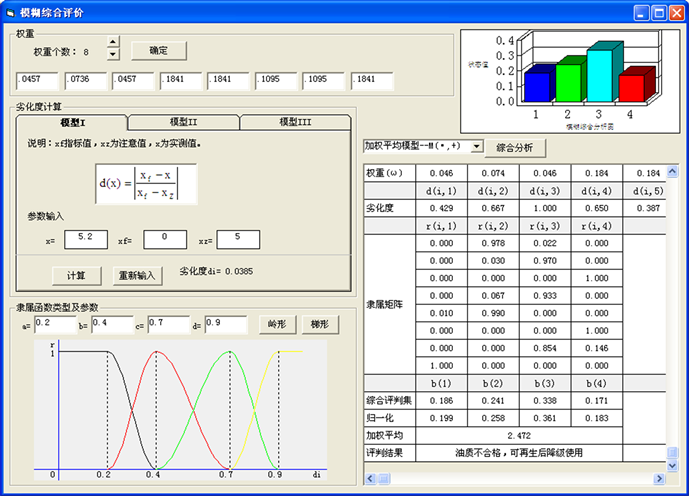﻿ 基于模糊综合评价的大型变压器油质SOH研究 Study on State of Health for Large Power Transformer Oil with Fuzzy Comprehensive Evaluation

Transmission and Distribution Engineering and Technology
Vol.06 No.03(2017), Article ID:22200,6 pages
10.12677/TDET.2017.63007

Study on State of Health for Large Power Transformer Oil with Fuzzy Comprehensive Evaluation

Yongchao Xue1, Rihong Xu2

1State Grid Pingdingshan Power Supply Company, Pingdingshan Henan

2School of Electrical Engineering, Wuhan University, Wuhan HubeiReceived: Sep. 9th, 2017; accepted: Sep. 22nd, 2017; published: Sep. 28th, 2017ABSTRACT

Rational use of transformer oils is inseparable from the evaluation of the health status of the oil quality. The transformer oil testing information is more, and part of the amount of factors exist uncertain characteristics. In this paper, based on the fuzzy comprehensive evaluation method, the mathematical model is constructed to evaluate the status of health for the transformer oil quality. The factor set and the evaluation set are built on the basis of this model. Then the ridge distribution is used as a membership function to establish the fuzzy relation matrix, and AHP method is used to quantify the weight. This paper presents a fuzzy comprehensive evaluation method to analyze oil qualitative health status, and the method is verified by examples. Examples analyses show that this method is reasonable and effective.

Keywords:Fuzzy Comprehensive Evaluation, State of Health, Power Transformer Oil

1国网平顶山供电公司，河南 平顶山

2武汉大学电气工程学院，湖北 武汉Copyright © 2017 by authors and Hans Publishers Inc.1. 引言

2. 模糊综合评价数学模型

$B=W\circ R=\left\{\left({b}_{1},{b}_{2},\cdots ,{b}_{m}\right)\right\}$ (1)

3. 油质模糊评价过程

3.1. 评判集、因素集的构成

$V=\left\{{v}_{1},{v}_{2},{v}_{3},{v}_{4}\right\}={\left\{\begin{array}{c}良好,\text{\hspace{0.17em}}可继续使用\\ 合格,\text{\hspace{0.17em}}应加强监测\\ 不良,\text{\hspace{0.17em}}处理后降级使用\\ 很坏,\text{\hspace{0.17em}}应更换\end{array}\right\}}^{\text{T}}$ (2)

3.2. 评价因素权重的确定

3.3. 评价指标劣化度

$d\left(x\right)=\frac{{x}_{f}-x}{{x}_{f}-{x}_{Z}}$ (3)

3.4. 模糊关系矩阵的确立

$r\left({d}_{i}\right)=\left\{\begin{array}{ll}0\hfill & \text{\hspace{0.17em}}\text{\hspace{0.17em}}{d}_{i}\le a\hfill \\ \frac{1}{2}+\frac{1}{2}\mathrm{sin}\frac{\text{π}}{b-a}\left[{d}_{i}-\frac{a+b}{2}\right]\hfill & a<{d}_{i}\le b\hfill \\ 1\hfill & b<{d}_{i}\le c\hfill \\ \frac{1}{2}-\frac{1}{2}\mathrm{sin}\frac{\text{π}}{d-c}\left[{d}_{i}-\frac{c+d}{2}\right]\hfill & c<{d}_{i}\le d\hfill \\ 0\hfill & \text{\hspace{0.17em}}\text{\hspace{0.17em}}{d}_{i}>d\hfill \end{array}$ (4)

3.5. 模糊综合评价

${b}_{j}=\underset{i=1}{\overset{n}{\sum }}\left({w}_{i}\cdot {r}_{ij}\right),\text{ }j=1,2,\cdots ,m$ (5)

4. 油质SOH评估示例Figure 1. The transformer oil SOH evaluation interface

5. 结论

Study on State of Health for Large Power Transformer Oil with Fuzzy Comprehensive Evaluation[J]. 输配电工程与技术, 2017, 06(03): 49-54. http://dx.doi.org/10.12677/TDET.2017.63007

1. 1. Phadungthin, R., Chaidee, E., Haema, J., et al. (2010) Analysis of Insulating Oil to Evaluate the Condition of Power Transformer. 7th Annual International Conference on Electrical Engineering/Electronics, Computer, Telecommunications and Information Technology, ECTI-CON 2010, Chiang Mai, 19-21 May 2010, 108-111.

2. 2. Da Silva, I.N., De Souza, A.N., Hossri, R.M.C., et al. (2000) Intelli-gent System Applied in Diagnosis of Transformer Oil. The 8h International Conference on Dielectric Materials, Measurements and Applications, Edinburgh, 17-21 September 2000, 330-334. https://doi.org/10.1049/cp:20000528

3. 3. 王平洋, 胡兆光. 模糊数学在电力系统中的应用[M]. 北京: 中国电力出版社, 1995.

4. 4. 马风才, 李霁坤, 张群. 基于三角形模糊数的DEA模型[J]. 数学的实践与认识, 2007, 37(11): 174-179.

5. 5. Jia, Z.Y., Wang, C.M., Huang, Z.W., et al. (2011) Evaluation Research of Regional Power Grid Companies’ Operation Capacity Based on Entropy Weight Fuzzy Comprehensive Model. Procedia Engineering, 15, 4626-4630. https://doi.org/10.1016/j.proeng.2011.08.869

6. 6. Cui, X., Liu, H.J., Ying, L.M. and Liao, Q.F. (2006) Multi-Level Fuzzy Com-prehensive Evaluation of Power Supply Service Quality. International Conference on Power System Technology, POWERCON2006, Chongqing, 22-26 October 2006, 1-6.

7. 7. Okubo, H., Kobayashi, S., Aoshima, Y., Takagi, H., et al. (1999) Electrical Insulation Di-agnostic Method and Maintenance Criteria for Oil-Immersed Power Transformers. IEEE International Conference on Conduction and Breakdown in Dielectric Liquids, ICDL, Nara, 372-377.

8. 8. IEEE Guide for Acceptance and Maintenance of Insulating Oil in Equipment. IEEE Std C57.106-2006, IEEE Transformers Committee, 2006.

9. 9. Mohamed, A., Wahab, A., Hamada, M.M. and Mo-hamed, A. (2011) Artificial Neural Network and Non-Linear Models for Prediction of Transformer Oil Residual Operating Time. Elec-tric Power Systems Research, 81, 219-227. https://doi.org/10.1016/j.epsr.2010.08.010

10. 10. 王莲芬, 许树柏. 层次分析法引论[M]. 北京: 中国人民大学出版社, 1992.